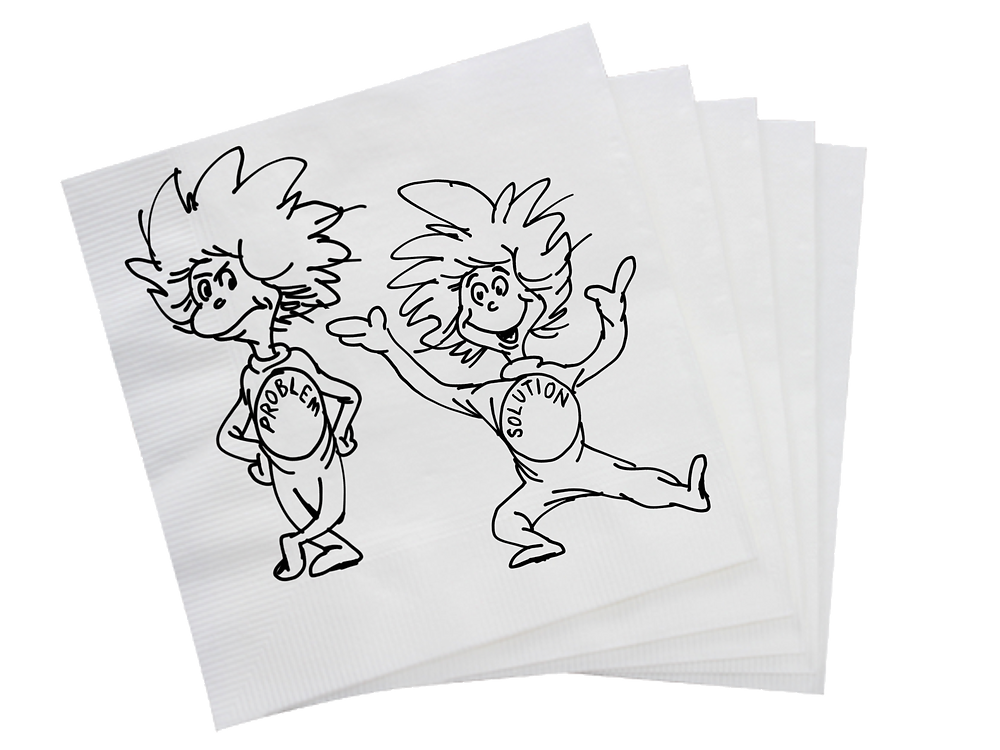# What exactly are Problems and Solutions?Or, “The differences between Thing One and Thing Two”

In this blog post, I will attempt to share some of my current thoughts and related ideas on “Problems and Solutions”.

Of course, everything is a thing.

But what makes this thing this thing? And what makes that thing that thing? And what makes this thing not that thing?

What exactly makes a given thing a particular thing?

What’s the difference?!

In biology, linguistics, philosophy, and logic, the difference is called the “differentia” – a distinguishing mark or feature. Differentiae help distinguish and determine one species from another, one definition from another, one idea from another, and one thing from another. Differentiae are the “essential (necessary) characteristics” that make a given thing a particular thing.

When trying to determine if it is reasonable to consider or call a given thing a particular thing, ask:

1. What are the “essential characteristics” of the particular thing?

2. Does the given thing possess all the “essential characteristics” of the particular thing?

If the given thing possesses all the “essential characteristics” of the particular thing, then it might be reasonable to consider and call the given thing “a particular thing”. But, if any “essential characteristics” of the particular thing are absent or different in the given thing, then it might not be reasonable to consider or call the given thing “a particular thing”.

An Example

Of course, rectangles and squares are things.

But what makes a rectangle a rectangle? And what makes a square a square? And what makes a rectangle not a square?

What exactly makes a given thing a rectangle or a square?

What’s the difference?!

A definition of “rectangle” is:

“a plane figure with four straight sides and four right angles”

Similarly, a definition of “square” is:

“a plane figure with four equal straight sides and four right angles”

While both rectangles and squares have “essential characteristics” of “a plane figure”, “four straight sides”, and “four right angles”, an additional “essential characteristic” of a square is “all four sides must be equal length”.

So, when trying to determine if it is reasonable to consider or call a given thing “a square”, ask:

1. What are the “essential characteristics” of a square?

• A plane figure

• Four straight sides

• Four right angles

• All four sides must be equal in length

2. Does the given thing possess all the “essential characteristics” of “a square”?

If the given thing possesses all the “essential characteristics” of a square, then it might be reasonable to consider and call the given thing “a square”. But, if any “essential characteristics” of a “square” are absent or different in the given thing, then it might not be reasonable to consider or call the given thing “a square”. Maybe it is a rectangle, or a triangle, or something else. But it probably isn’t a square.

Applying this idea to Problems and Solutions

Of course, problems and solutions are things, too.

But what makes a problem a problem? And what makes a solution a solution? And what makes a problem not a solution?

What exactly makes a given thing a problem or a solution?

What’s the difference?!

A general definition of “problem” is simply:

“an undesirable state that differs from a desirable state/goal”.

So, when trying to determine if it is reasonable to consider or call a given thing “a problem”, ask:

1. What are the “essential characteristics” of a problem?

• an undesired state

• different than some desired state/goal

2. Does the given thing possess all the “essential characteristics” of “a problem”?

If the given thing possesses all the “essential characteristics” of a problem, then it might be reasonable to consider and call the given thing “a problem”. But, if any “essential characteristics” of a problem are absent or different in the given thing, then it might not be reasonable to consider or call the given thing “a problem”. Maybe it is a challenge, or an opportunity, or something else. But it probably isn’t a problem.

Similarly, a general definition of “solution” is:

“a desirable state/goal that differs from an undesirable state”.

When trying to determine if it is reasonable to consider or call a given thing “a solution”, ask:

1. What are the “essential characteristics” of a solution?

• a desired state/goal

• different than some undesired state

2. Does the given thing possess all of the “essential characteristics” of “a solution”?

If the given thing possesses all of the “essential characteristics” of a solution, then it might be reasonable to consider and call the given thing “a solution”. But, if any “essential characteristics” of a solution are absent or different in the given thing, then it might not be reasonable to consider or call the given thing “a solution”. Maybe it is a result, or an explanation, or something else. But it probably isn’t a solution.

Feelings

Note that in both cases, “desire” – a strong feeling of wanting – and its converse are important and essential elements of problems and solutions. If a given thing doesn’t affect positive or negative feelings, then perhaps it isn’t a problem or solution, at all.

And, of course, “desire” is relative. That is, desire describes a particular feeling and relationship between someone and something at some time. A problem to me might not be a problem to you. And a solution to me might not be a solution to you. And a solution to us now might be a problem to us later!

Which all leads to my favorite joke on this topic:

Q: What’s the fastest way to solve a problem?

A: Tell someone that doesn’t care.

In My Experience, deeper understanding of problems and solutions - through consideration of “differentia”, their “essential characteristics”, and the importance of subjective feelings - has helped me better identify, comprehend, and address them both.

I examine and explore "Problems and Solutions" in my talk, “The Hidden Requirements”.

92 views